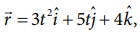Home | | Physics 11th std | Solved Example Problems for Accelerated Motion

# Solved Example Problems for Accelerated Motion

Physics : Kinematics : Accelerated Motion

Solved Example Problems for Accelerated Motion

### Example 2.31

A velocity–time graph is given for a particle moving in x direction, as belowa) Describe the motion qualitatively in the interval 0 to 55 s .

b) Find the distance and displacement travelled from 0 s  to 40 s .

c) Find the acceleration at t = 5 s  and at t 20 s

### Solution

a) From O to A: (0 s  to 10 s )

At t = 0 s  the particle has zero velocity.

At t > 0, particle has positive velocity and moves in the positive x direction.

From 0 s   to 10 s   the slope (dv/dt) positive, implying the particle is accelerating. Thus the velocity increases during this time interval.

From A to B: (10 s  to 15 s )

From 10 s   to 15 s   the velocity stays constant at 60 m s-1. The acceleration is 0 during this period. But the particle continues to travel in the positive x-direction.

From B to C : (15 s  to 30 s )

From the 15 s   to 30 s   the slope is negative, implying the velocity is decreasing. But the particle is moving in the positive x direction. At t = 30 s   the velocity becomes zero, and the particle comes to rest momentarily at t = 30 s .

From C to D: (30 s  to 40 s )

From 30 s  to 40 s  the velocity is negative. It implies that the particle starts to move in the negative x direction. The magnitude of velocity increases to a maximum 40 m s-1

From D to E: (40 s  to 55 s )

From 40 s   to 55 s   the velocity is still negative, but starts increasing from –40 m s-1 At t = 55 s  the velocity of the particle is zero and particle comes to rest.

(b) The total area under the curve from 0 s  to 40 s  will give the displacement. Here the area from O to C represents motion along positive x–direction and the area under the graph from C to D represents the particle's motion along negative x–direction.

The displacement travelled by the particle from 0 s to 10 s = 1/2 × 10 × 60 = 300m

The displacement travelled from 10 s to 15 s = 60 × 5 = 300 m

The displacement travelled from 15 s to 30 s =1/2 × 15 × 60 = 450m

The displacement travelled from 30 s to 40 s = 1/2 × 10 × (-40) = -200m.

Here the negative sign implies that the particle travels 200 m in the negative x direction.300 m + 300 m + 450 m − 200 m = +850 m.

Thus the particle's net displacement is along the positive x-direction.

The total distance travelled by the

particle from 0 s to 40 s = 300 + 300 + 450 + 200 = 1250 m.

(c) The acceleration is given by the slope in the velocity-time graph. In the first 10 seconds the velocity has constant slope (constant acceleration). It implies that the acceleration a is from v1 = 0 to v2 = 60 m s-1.Next, the particle has constant negative  slope from 15 s to 30 s . In this case v2=0 and v1=60m s-1. Thus the acceleration at t = 20 s is given by a = (0-60)/(30-15) = 4 m s-2. Here the negative sign implies that the particle has negative acceleration.

### Example 2.32

If the position vector of the particle is given byFind the

a. The velocity of the particle at t = 3 s

b. Speed of the particle at t = 3 s

c. acceleration of the particle at time t = 3 s

### Solution### Example 2.33

An object is thrown vertically downward. What is the acceleration experienced by the object?

### Solution

We know that when the object falls towards the Earth, it experiences acceleration due to gravity g = 9.8 m s-2 downward. We can choose the coordinate system as shown in the figure.The acceleration is along the negative y direction.Study Material, Lecturing Notes, Assignment, Reference, Wiki description explanation, brief detail
11th Physics : UNIT 2 : Kinematics : Solved Example Problems for Accelerated Motion |